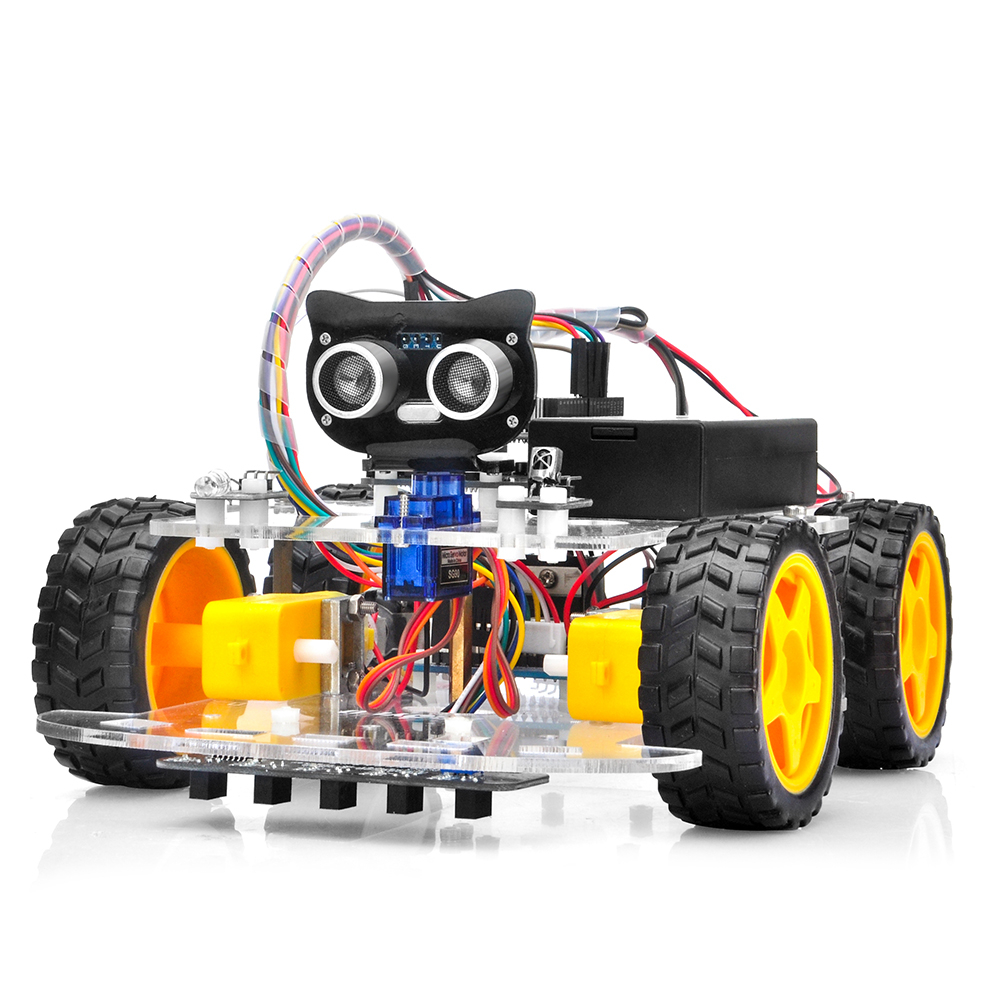# Joystick to Differential Drive (Python)

As I’m now working on a Raspberry Pi Zero W based robot, doing my first test on Python programming, I needed to port the function created in my previous post for Arduino (c++). This is the same but written on python

Posted by Simar Mann Singh on 03 May, 2022

As I’m now working on a Raspberry Pi Zero W based robot, doing my first test on Python programming, I needed to port the function created previously in this article for Arduino (c++).

This time though, I changed its name, and added the capability to provide the limits for the input and the output on any range you need it. I’ll explain why Let’s suppose you have an input that is like a joystick, so the x, and y values provided by the joystick will vary between -1 and 1, as can be seen in the diagram.Joystick output requirements

We need to convert this pair of values, to something we can use to drive a Differential drive (aka tank drive) robot. This is what we need the bot to behave like:differential drive

To achieve this, is that I made this function. Here you can check it:

```def joystickToDiff(x, y, minJoystick, maxJoystick, minSpeed, maxSpeed): # If x and y are 0, then there is not much to calculate... if x == 0 and y == 0: return (0, 0) # First Compute the angle in deg # First hypotenuse z = math.sqrt(x * x + y * y) # angle in radians rad = math.acos(math.fabs(x) / z) # and in degrees angle = rad * 180 / math.pi # Now angle indicates the measure of turn # Along a straight line, with an angle o, the turn co-efficient is same # this applies for angles between 0-90, with angle 0 the coeff is -1 # with angle 45, the co-efficient is 0 and with angle 90, it is 1 tcoeff = -1 + (angle / 90) * 2 turn = tcoeff * math.fabs(math.fabs(y) - math.fabs(x)) turn = round(turn * 100, 0) / 100 # And max of y or x is the movement mov = max(math.fabs(y), math.fabs(x)) # First and third quadrant if (x >= 0 and y >= 0) or (x < 0 and y < 0): rawLeft = mov rawRight = turn else: rawRight = mov rawLeft = turn # Reverse polarity if y < 0: rawLeft = 0 - rawLeft rawRight = 0 - rawRight # minJoystick, maxJoystick, minSpeed, maxSpeed # Map the values onto the defined rang rightOut = map(rawRight, minJoystick, maxJoystick, minSpeed, maxSpeed) leftOut = map(rawLeft, minJoystick, maxJoystick, minSpeed, maxSpeed) return (rightOut, leftOut) def map(v, in_min, in_max, out_min, out_max): # Check that the value is at least in_min if v < in_min: v = in_min # Check that the value is at most in_max if v > in_max: v = in_max return (v - in_min) * (out_max - out_min) // (in_max - in_min) + out_min```

I added as many comments as I could, trying to make everything clear.

So, what are the differences with the Arduino version?

The name: just because I think this is more accurate to the purpose of the function

It returns a tuple: So you just get the right and left speeds on it

The joystick and wheel speed limits

This change is the most important. In reality, I´m not using a joystick to control the robot, but my cellphone´s accelerometer, so, thanks to this parameters, I can reconfigure the limits of the input.

In case of a joystick might be -1 to 1, but in case of the accelerometer, it goes from -9.8 to 9.8 (the gravity)

Also, I wanted the output to be configurable with the minSpeed, maxSpeed parameters. This allows me to just get the range of values I need for my servo controller (in my case -4095 to 4095, but you can set whatever speed limits you want there, and the output will be scaled accordingly)

#### What about that map function?

If you are an Arduino programmer, you might know it already, since I port it from there. It is used to map an input value from the input range limits, to the output range limits.

As I´m not aware of it existing in python, I just added to my utils functions.

The only difference with the Arduino version, is that I added a constraint to the value, so it cannot be smaller or bigger than the input range specified.

I hope this makes sense, and if it doesn’t, please let me know. I promise I’ll do my best to make it more clear.